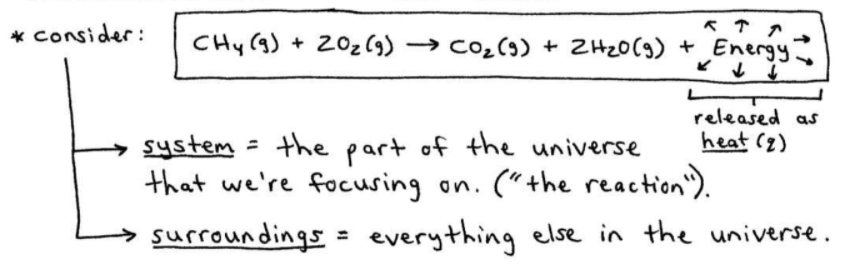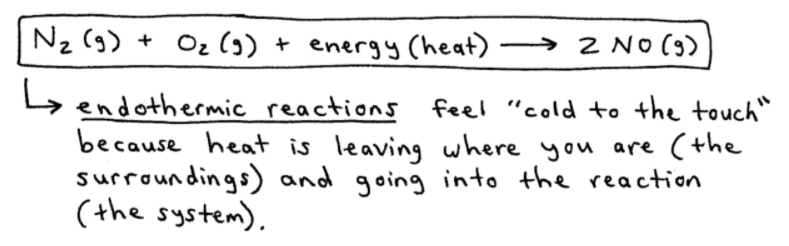# S6E1 - Potential vs. Kinetic Energy, and Heat

## The Nature of Energy

Energy  =  the capacity to do work (w) or to produce heat (q).

The concept of "energy" is familiar to us, but energy is a bit difficult to define.

The total energy of the universe is conserved.

It can be converted from one form to another, but it can never be created nor destroyed.

==========

## Types of Energy with Examples

There are many types of energy, but two important ones for 1st year Chemistry are defined below...

1. Potential Energy  =  "stored energy."

It's the energy associated with the position or composition of an object.

### Potential Energy Examples:

➞  water behind a dam

➞  gasoline in a parked car

➞  ball at the top of a hill

➞  chemical bonds in the reactants

2. Kinetic Energy - "the energy of motion"

It's the energy that depends on the moving object's mass and velocity.  KE = (1/2)mv2### Kinetic Energy Examples:

➞  water flowing in a river

➞  driving a moving car

➞  ball rolling down a hill

➞  chemical bonds actively breaking or forming

==========

## The Difference Between Temperature and Heat

Temperature (T)  =  a measure of the random motions of the particles in a substance.

Heat (q)  =  involves the transfer of energy between two objects due to a temperature difference.

Heat (q)  =  flows spontaneously from a hot object to a cooler one.

Heat (q)  =  does not represent a "substance" contained by an object.

==========

## The Transfer of Chemical Energy.

#1  Consider the following reaction:

CH4(g)  +  2O2(g)  ➞  CO2(g)  +  2H2O(g)  + EnergyAs you can see in the image above,

The "system" (in the "box") is the reaction - the part of the universe we're focusing on.

➞   system - reactants and products in the reaction

The "surroundings" are (quite literally) everything else, including the person running the experiment :-)

➞   surroundings - reaction container / vessel, the lab, etc...

==========

## Exothermic vs. Endothermic Reactions

#1  In the sample reaction above, energy is released as heat.

It's an exothermic reaction.

In an exothermic reaction, some of the potential energy stored in the chemical bonds is being converted to thermal energy via heat.

So, energy is released to the surroundings as heat.

Therefore, exothermic reactions feel "hot to the touch."

----------

Oppositely,

#2  Consider the following reaction:

N2(g)  +  O2(g)  + Energy  ➞  2NO(g)

When heat flows into the system, the process is an endothermic reaction:Endothermic reactions feel "cold to the touch" because heat is leaving where you are (the surroundings) and going into the reaction (the system).

==========

## 2 key definitions before we move on...

a.  Thermodynamics  =  the study of energy and its interconversions.

b.  First Law of Thermodynamics  =  the total energy of the universe is constant.

==========

The next post in this section, SECTION 6 - Thermochemistry,

discusses how to calculate the Energy (E) of a System, Work (w), and Heat (q) ...